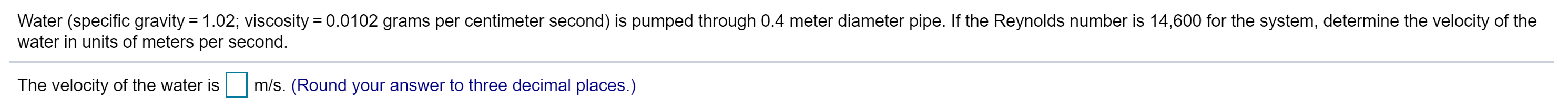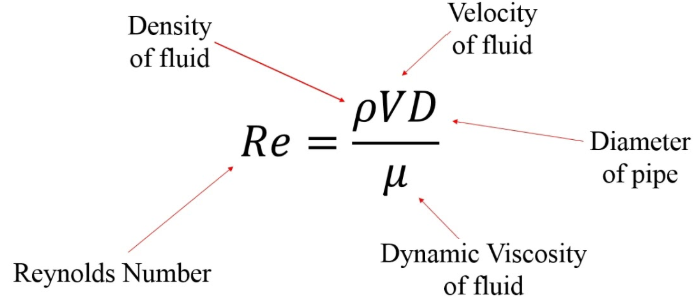# Water (specific gravity 1.02; viscosity 0.0102 grams per centimeter second) is pumped through 0.4 meter diameter pipe. If the Reynolds number is 14,600 for the system, determine the velocity of thewater in units of meters per second.The velocity of the water ism/s. (Round your answer to three decimal places.) Velocityof fluidDensityof fluidpVDReDiameterof pipeиDynamic Viscosityof fluidReynolds Number

Question
56 views

What is the velocity of water given the following?help_outlineImage TranscriptioncloseWater (specific gravity 1.02; viscosity 0.0102 grams per centimeter second) is pumped through 0.4 meter diameter pipe. If the Reynolds number is 14,600 for the system, determine the velocity of the water in units of meters per second. The velocity of the water is m/s. (Round your answer to three decimal places.) fullscreenhelp_outlineImage TranscriptioncloseVelocity of fluid Density of fluid pVD Re Diameter of pipe и Dynamic Viscosity of fluid Reynolds Number fullscreen
check_circle

Step 1

Given:

Diameter of pipe = 0.4 m

Dynamic viscosity of water = 0.01 kg m/s

Density of water = 997 kg/m3

Reynold’s number = 14,600

Step 2

Plug in the values in t...

### Want to see the full answer?

See Solution

#### Want to see this answer and more?

Solutions are written by subject experts who are available 24/7. Questions are typically answered within 1 hour.*

See Solution
*Response times may vary by subject and question.
Tagged in

### Fluid Mechanics